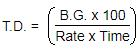Courses

# Test: Banker's Discount- 1

## 10 Questions MCQ Test UPSC CSAT: Logical Reasoning (LR) & Data Interpretation (DI) | Test: Banker's Discount- 1

Description
This mock test of Test: Banker's Discount- 1 for CAT helps you for every CAT entrance exam. This contains 10 Multiple Choice Questions for CAT Test: Banker's Discount- 1 (mcq) to study with solutions a complete question bank. The solved questions answers in this Test: Banker's Discount- 1 quiz give you a good mix of easy questions and tough questions. CAT students definitely take this Test: Banker's Discount- 1 exercise for a better result in the exam. You can find other Test: Banker's Discount- 1 extra questions, long questions & short questions for CAT on EduRev as well by searching above.
QUESTION: 1

### Q. The banker's discount on a bill due 4 months hence at 15% is Rs. 420. What is the true discount?

Solution: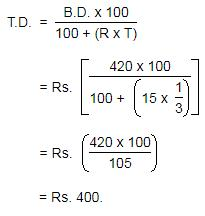QUESTION: 2

### The banker's discount on a certain amount due 2 years hence is 11/10 of the true discount. What is the rate percent?

Solution: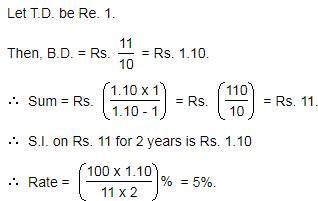QUESTION: 3

### The present worth of a sum due sometime hence is Rs. 576 and the banker's gain is Rs. 16. The true discount is:

Solution: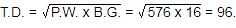QUESTION: 4

What is the banker's discount if the true discount on a bill of Rs.540 is Rs.90 ?

Solution: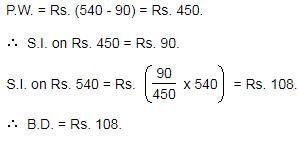QUESTION: 5

A bill for Rs. 3000 is drawn on 14th July at 5 months. It is discounted on 5th October at 10%. What is the Banker's Discount?

Solution:

F = Rs. 3000
R = 10%
Date on which the bill is drawn = 14th July at 5 months
Nominally Due Date = 14th December
Legally Due Date = 14th December + 3 days = 17th December
Date on which the bill is discounted = 5th October
Unexpired Time
= [6th to 31th of October] + [30 Days in November] + [1th to 17th of December]
= 26 + 30 + 17
= 73 Days
= 73/365year = 1/5year
Banker's Discount = Simple Interest on the face value of the bill for unexpired time = FTR/100
= (3000×1/5×10)/100
= 30×1/5×10
= Rs.60

QUESTION: 6

The bankers discount and the true discount of a sum at 10% per annum simple interest for the same time are Rs.100 and Rs.80 respectively. What is the sum and the time?

Solution:

BD = Rs.100
TD = Rs.80
R = 10%
F = BD×TD/(BD−TD)
= (100×80)/(100−80)
= (100×80)/20
= Rs.400
BD = Simple Interest on the face value of the bill for unexpired time = FTR/100
⇒ 100 = (400×T×10)/100
⇒ 100 = 4×T×10
⇒ 10 = 4×T
⇒ T = 10/4
= 2.5years

QUESTION: 7

The banker's gain on a sum due 6 years hence at 12% per annum is Rs. 540. What is the banker's discount?

Solution: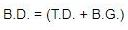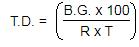QUESTION: 8

The present worth of a certain bill due sometime hence is Rs. 1296 and the true discount is Rs. 72. What is the banker's discount?

Solution: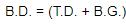and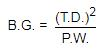QUESTION: 9

The banker's discount of a certain sum of money is Rs. 36 and the true discount on the same sum for the same time is Rs. 30. What is the sum due?

Solution: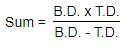QUESTION: 10

The banker's gain on a bill due 1 year hence at 10% per annum is Rs. 20. What is the true discount?

Solution: